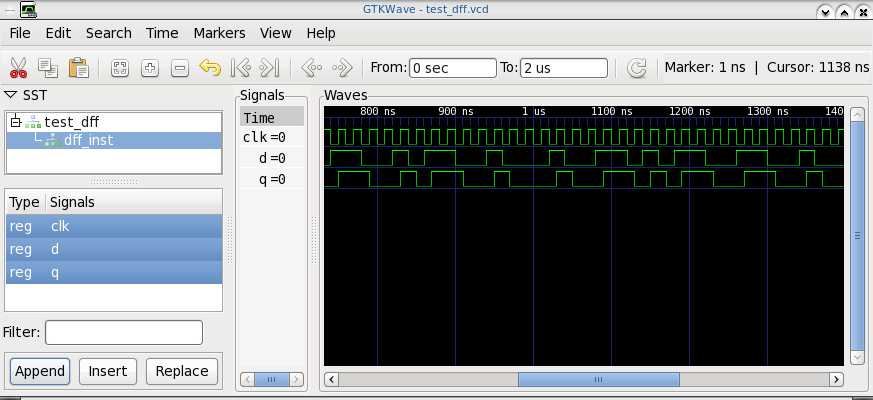news

MyHDL, a Python hardware description and verification language is now available for Fedora/RHEL. Install it using:

``\$ sudo yum install python-myhdl``

A simple example of a D flip-flop is given below:

``````#!/usr/bin/python

from myhdl import *
from random import randrange

def dff(q, d, clk):
@always(clk.posedge)
def logic():
q.next = d

return logic

def test_dff():
q, d, clk = [Signal(bool(0)) for i in range(3)]

dff_inst = dff(q, d, clk)

@always(delay(10))
def clkgen():
clk.next = not clk

@always(clk.negedge)
def stimulus():
d.next = randrange(2)

return dff_inst, clkgen, stimulus

def simulate(timesteps):
tb = traceSignals(test_dff)
sim = Simulation(tb)
sim.run(timesteps)

simulate(2000)``````

You can run it using:

``\$ python test.py``

The generated test_dff.vcd can be viewed in GTKWave:You can also generate Verilog code using the toVerilog() function. For example:

``````def convert():
q, d, clk = [Signal(bool(0)) for i in range(3)]
toVerilog(dff, q, d, clk)

convert()``````

The generated Verilog code looks like:

```````timescale 1ns/10ps

module dff (
q,
d,
clk
);

output q;
reg q;
input d;
input clk;

always @(posedge clk) begin: DFF_LOGIC
q &lt;= d;
end

endmodule``````

Refer their wiki for more documentation.